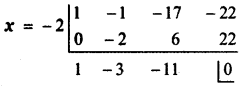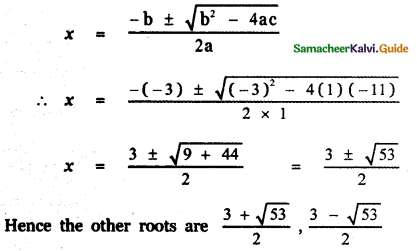Tamilnadu State Board New Syllabus Samacheer Kalvi 11th Maths Guide Pdf Chapter 2 Basic Algebra Ex 2.6 Text Book Back Questions and Answers, Notes.

## Tamilnadu Samacheer Kalvi 11th Maths Solutions Chapter 2 Basic Algebra Ex 2.6

Question 1.
Find the zeros of the polynomial function f(x) = 4x2 – 25
Given f(x) = 4x2 – 25
To find the zeors of f(x), put f(x) = 0
∴ 4x2 – 25 = 0
⇒ 4x2 = 25
⇒ x2 = $$\frac{25}{4}$$
⇒ x = ±$$\sqrt{\frac{25}{4}}$$ = ±$$\frac{5}{2}$$
Hence the zeros of f(x) are $$-\frac{5}{2}, \frac{5}{2}$$Question 2.
If x = – 2 is one root of x3 – x2 – 17x = 22, then find the other roots of equation.
Let f(x) = x3 – x2 – 17x – 22 = 0 —– (1)
Given that x = – 2 is a root of f(x).
∴ x + 2 is a factor of f (x)
Using synthetic divisionComparing equation (1) with the equation ax2 + bx + c = 0 we have
a = 1, b = – 3 , c = – 11Question 3.
Find the real roots of x4 = 16.
x4 = 16
⇒ x4 – 16 = 0
(i.e.,) x4 – 42 = 0
⇒ (x2 + 4)(x2 – 4) = 0
x2 + 4 = 0 will have no real roots
so solving x2 – 4 = 0
x2 = 4Question 4.
Solve (2x + 1)2 – (3x + 2)2 = 0
x = – $$\frac{3}{5}$$ or x = – 1
∴ Solution set is { – 1, $$\frac{3}{5}$$}